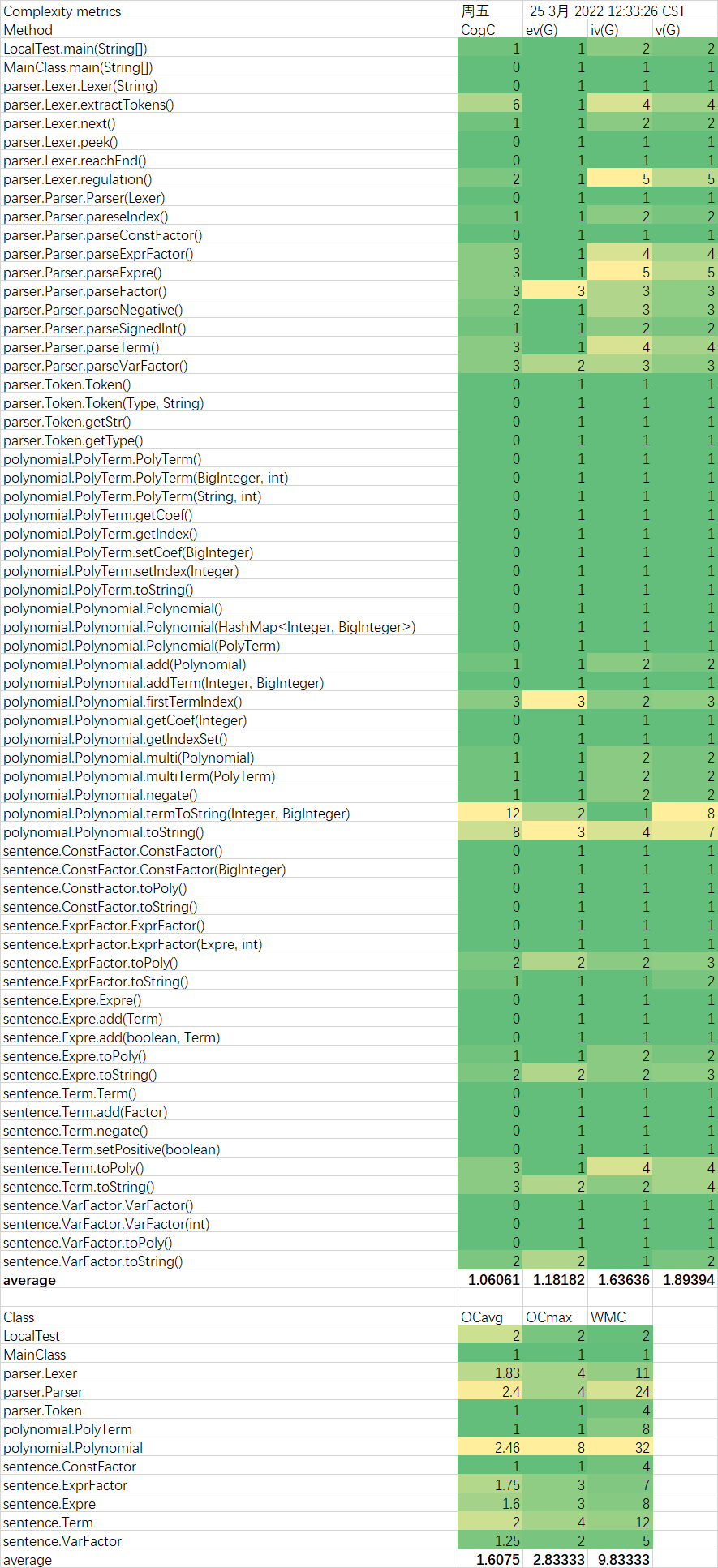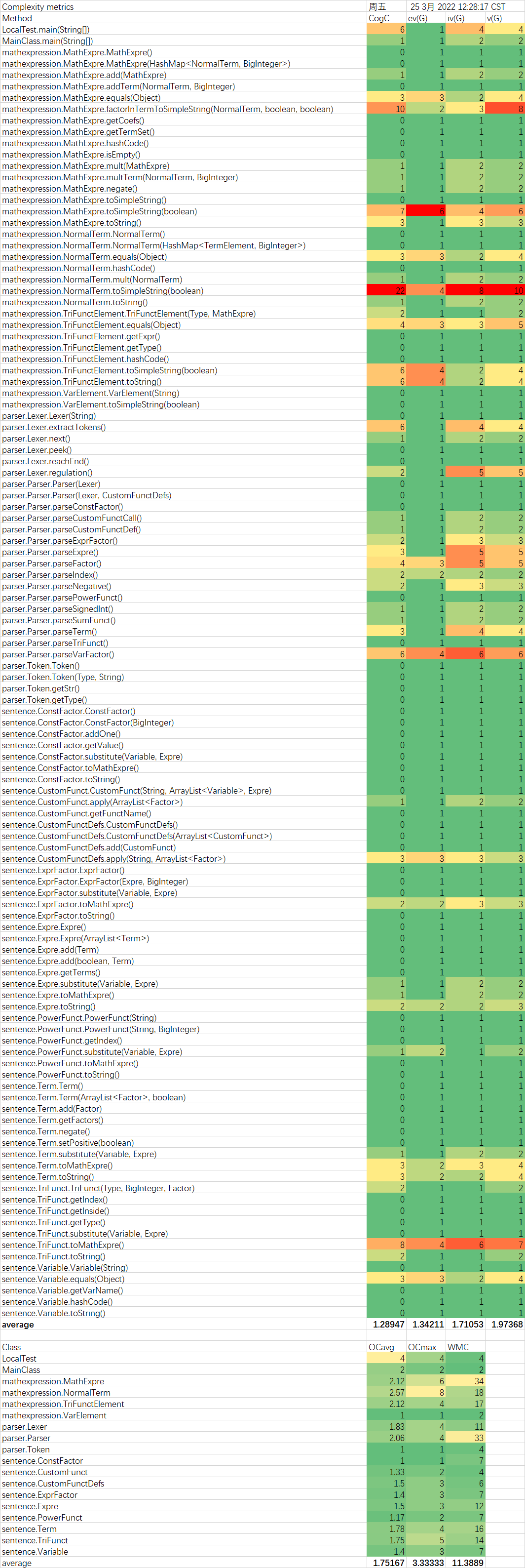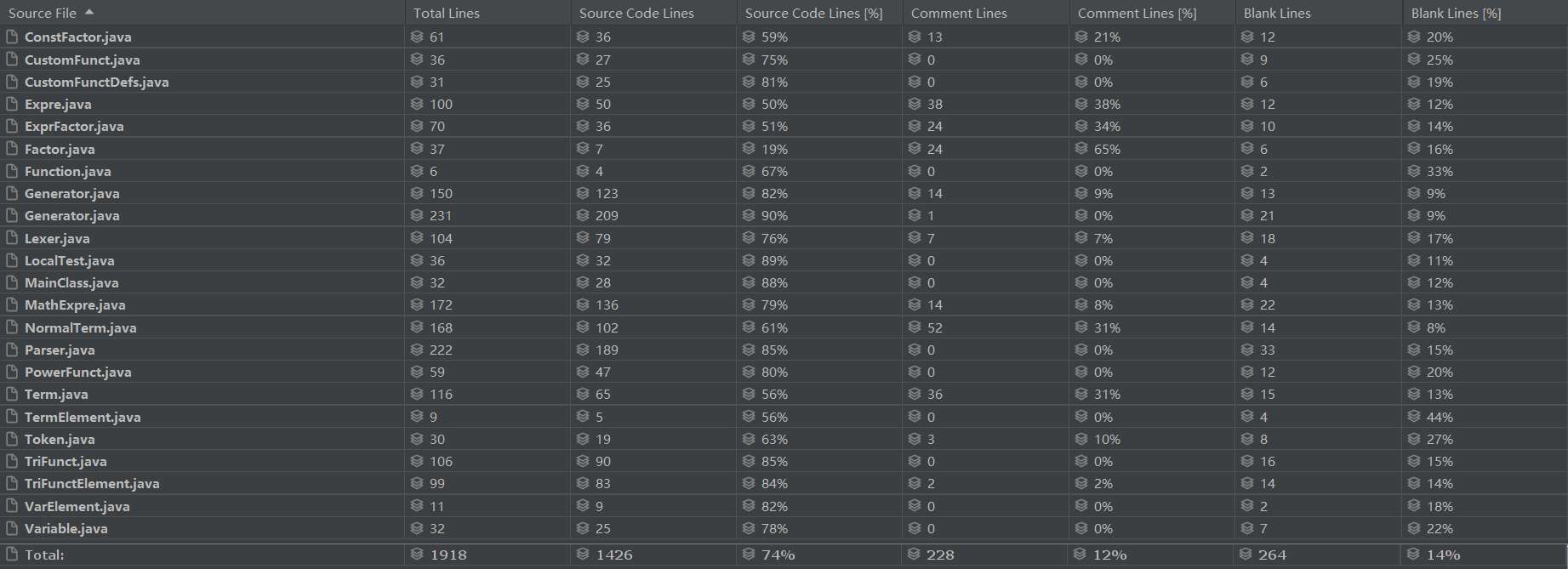# 第一单元总结

## 作业分析

\begin{aligned} Expre &\rightarrow [+|-]Term \lbrace (+|-)Term \rbrace \\ Term &\rightarrow [+|-]Factor \lbrace * Factor \rbrace \\ Factor &\rightarrow ConstFactor\;|\;ExprFactor\;|\;VarFactor\\ ConstFactor &\rightarrow SignedInt \\ ExprFactor &\rightarrow (Expre)[Index]\\ VarFactor &\rightarrow PowerFunct \;|\; TriFunct \;|\; SumFunct \;|\; CustomFunctCall \\ PowerFunct &\rightarrow Var \;|\; i \;\; [Index] \\ TriFunct &\rightarrow sin(Factor) \;|\; cos(Factor) \;\; [Index] \\ SumFunct &\rightarrow sum(i, ConstFactor, ConstFactor, Factor) \\ CustomFunctCall &\rightarrow FunctName(Factor\lbrace , Factor \rbrace ) \\ CustomFunctDef &\rightarrow FunctName(Var \lbrace ,Var\rbrace ) = Expre \\ Index &\rightarrow **[+]Int\\ Int &\rightarrow (0|1|2|3|4|5|6|7|8|9)\lbrace 0|1|2|3|4|5|6|7|8|9 \rbrace \\ SignedInt &\rightarrow [+|-]Int\\ Var &\rightarrow x\;|\;y\;|\;z\\ FunctName &\rightarrow f\;|\;g\;|\;h\\ \\ \end{aligned}

## 整体架构

### 架构思路

$\sum_j k_j \prod_i f_{j,i}^{p_{j,i}},\;\;f_{j,i}=x\text{或}sin(Expre)\text{或}cos(Expre)$

### 整体架构

UML类图如下：1. parser: 按文法解析输入
2. sentence: 输入表达式，按给定的结构层次存储
3. mathexpression: 数学表达式，实现了加法、乘法运算

parser包中，使用递归下降法，解析输入的字符串。其中的lexer类用于识别字符串中的不同组件，parser类中有一系列解析方法，用于递归

sentence包中的各个类均是直接按照输入字符串的文法定义进行构造的，逻辑上只是按结构储存输入的字符串，而非其对应的数学意义上的表达式，这也是该包命名为"sentence"而非"expression"的原因。

mathexpression包主要描述了数学表达式。顶层为MathExpre，该表达式是若干个NormalTerm的线性组合。每个NormalTerm包含了若干个三角函数或变元的幂，它们统一实现了TermElement接口。再NormalTerm中使用HashMap储存底数与幂的关系，在MathExpre中依然使用HashMap储存NormalTerm及其系数的关系。即使用两层的HashMap来存储一个数学表达式。在MathExpre中，实现了若干加法、乘法的方法。

#### 这种架构是如何拆括号的？

sentence包内部的所有类都实现了一个toMathExpre方法，该方法将当前对象转换为数学表达式，并返回一个mathexpression.MathExpre类的数据。

#### 这种架构的优缺点是什么？

1. 输入表达式可能有多层嵌套，但相邻的层次间的逻辑关系简单（每个表达式都是若干个项的，每个项都是若干因子的
2. 计算的最终结果可以有一致的结构

### 其他架构的讨论

|- Factor(Interface)
|- ExpreFactor
|- Term
|- ConstFactor
|- TriFunct
|- PowerFunct


• 表达式可以认为是一个系数为1的表达式因子
• 项可以认为是一个表达式，而表达式可以认为是表达式银子

## 程序结构分析

### 第一次作业

#### 代码规模#### 复杂度分析### 第二次作业

#### 代码规模#### 复杂度分析### 第三次作业

#### 代码规模#### 复杂度分析#### 静态分析## 测试与bug

### 范式不唯一

$1 * x^1 \\ 1 * x^1 * sin^0(x^333333)\\ 1 * x^1 * cos^0(x^0) \\ 1 * x^1 * sin^0(x) * cos^0(x) * sin^0(x^2) * cos^0(x^2) * sin^0(x^3) * cos^0(x^3)$

### 变量名冲突

public Expre apply(ArrayList<Factor> tars) {
Expre ans = definition;
for (int i = 0; i < vars.size(); ++i) {
ArrayList<Term> terms = new ArrayList<>();
ArrayList<Factor> factors = new ArrayList<>();
Expre target = new Expre(terms);
ans = ans.substitute(vars.get(i), target);
}
return ans;
}


1
f(y, x) = y + x
f(x**2, x**5)


## 心得体会

1. 打开冰箱门
2. 观察冰箱内部第一层
3. 判断该层是否有空余位置
• 若有，则转到4
• 否则，观察冰箱的下一层，转到3
4. 将物品放入该层
5. 关闭冰箱门

posted on 2022-03-25 20:55  StyWang  阅读(41)  评论(1编辑  收藏  举报